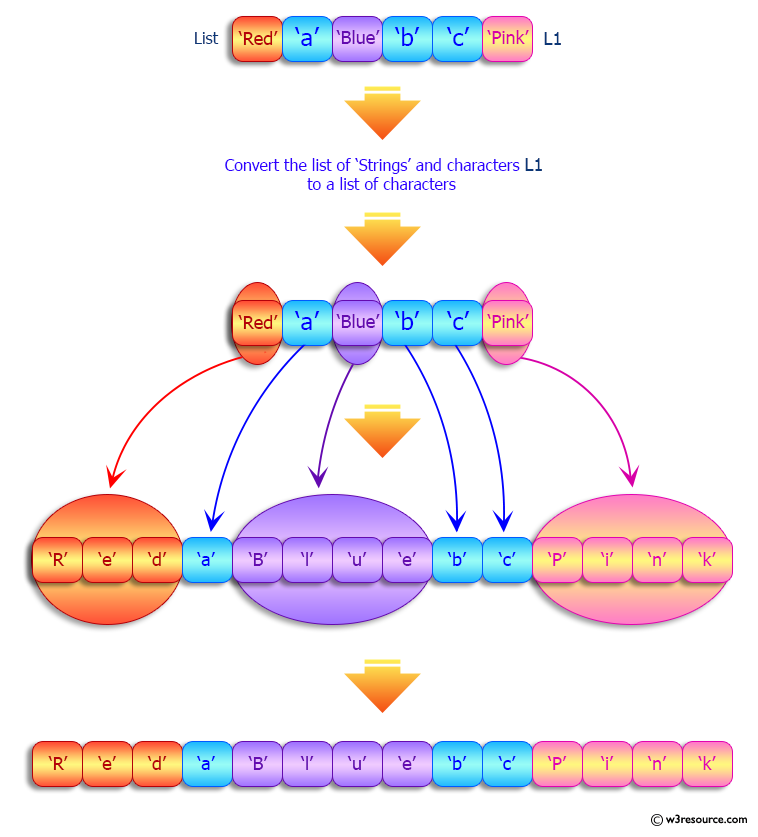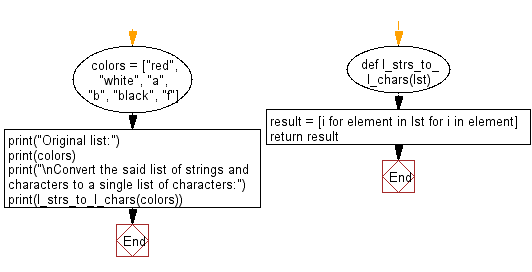﻿ Python: Convert a given list of strings and characters to a single list of characters - w3resource# Python: Convert a given list of strings and characters to a single list of characters

## Python List: Exercise - 169 with Solution

Write a Python program to convert a given list of strings and characters to a single list of characters.

Sample Solution:

Python Code:

``````def l_strs_to_l_chars(lst):
result = [i for element in lst for i in element]
return result

colors = ["red", "white", "a", "b", "black", "f"]
print("Original list:")
print(colors)
print("\nConvert the said list of strings and characters to a single list of characters:")
print(l_strs_to_l_chars(colors))
```
```

Sample Output:

```Original list:
['red', 'white', 'a', 'b', 'black', 'f']

Convert the said list of strings and characters to a single list of characters:
['r', 'e', 'd', 'w', 'h', 'i', 't', 'e', 'a', 'b', 'b', 'l', 'a', 'c', 'k', 'f']
```

Pictorial Presentation:Flowchart:## Visualize Python code execution:

The following tool visualize what the computer is doing step-by-step as it executes the said program:

Python Code Editor:

Have another way to solve this solution? Contribute your code (and comments) through Disqus.

What is the difficulty level of this exercise?

Test your Python skills with w3resource's quiz

﻿

## Python: Tips of the Day

Floor Division:

When we speak of division we normally mean (/) float division operator, this will give a precise result in float format with decimals.

For a rounded integer result there is (//) floor division operator in Python. Floor division will only give integer results that are round numbers.

```print(1000 // 300)
print(1000 / 300)```

Output:

```3
3.3333333333333335```